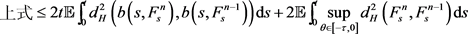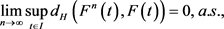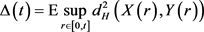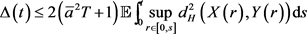﻿ 一类泛函集值随机微分方程的解

# 一类泛函集值随机微分方程的解The Solution of a Type of Functional Set-Valued Stochastic Differential Equation

Abstract: In this paper, we shall introduce set-valued and its properties, and discuss the functional set-valued stochastic differential equation. When the initial value is given, we shall prove the existence and uniqueness of solution of the type of functional set-valued stochastic differential equation.

1. 前言及基础不等式

${d}_{H}\left(A,B\right)=\mathrm{max}\left\{{\mathrm{sup}}_{a\in A}d\left(a,B\right),{\mathrm{sup}}_{b\in B}d\left(b,A\right)\right\}$

${d}_{H}\left(A+B,C+D\right)\le {d}_{H}\left(A,C\right)+{d}_{H}\left(B,D\right)$ (1)

${d}_{H}^{2}\left({\int }_{0}^{t}F\left(s,\omega \right)\text{d}s,{\int }_{0}^{t}G\left(s,\omega \right)\text{d}s\right)\le t{\int }_{0}^{t}{d}_{H}^{2}\left(F\left(s,\omega \right),G\left(s,\omega \right)\right)\text{d}s$ (2)

$\tau >0$$C\left(\left[-\tau ,0\right];K\left({ℝ}^{d}\right)\right)$ 是所有的从 $\left[-\tau ,0\right]$$K\left({ℝ}^{d}\right)$ 的Hausdorff距离连续的集值映射的全体。集值泛函随机微分方程

$\text{d}F\left(t\right)=b\left(t,{F}_{t}\right)\text{d}t+\sigma \left(t,{F}_{t}\right)\text{d}G\left(t\right),\text{\hspace{0.17em}}\text{\hspace{0.17em}}t\in I$

2. 初值问题

$F\left(0\right)=\xi =\left\{\xi \left(\theta \right):-\tau \le \theta \le 0\right\}$${\mathcal{A}}_{0}$ 可测的 $C\left(\left[-\tau ,0\right];K\left({ℝ}^{d}\right)\right)$ -值的随机映射，且满足 $\mathbb{E}\left({\mathrm{sup}}_{\theta \in \left[-\tau ,0\right]}{‖\xi ‖}_{K}^{2}\right)<\infty$

3. 方程解的存在唯一性证明

$F\left(t\right)=\xi \left(0\right)+\left(L\right){\int }_{0}^{t}b\left(s,{F}_{s}\right)\text{d}s+\left(M\right){\int }_{0}^{t}\sigma \left(s,{F}_{s}\right)\text{d}G\left(s\right),\text{\hspace{0.17em}}\text{\hspace{0.17em}}t\in I$

1) 线性增长条件：对 $\forall \phi \in C\left(\left[-\tau ,0\right];{K}_{k}\left({ℝ}^{d}\right)\right)$$\exists$ 常数a，s.t.对 $\forall t\in I$

${‖b\left(t,\phi \right)‖}_{K}^{2}+{‖\sigma \left(t,\phi \right)‖}^{2}\le {a}^{2}\left(1+\underset{\theta \in \left[-\tau ,0\right]}{\mathrm{sup}}{‖\phi \left(\theta \right)‖}_{K}^{2}\right)$

2) Lipschitz连续条件：对 $\forall \phi ,\varphi \in C\left(\left[-\tau ,0\right];{K}_{k}\left({ℝ}^{d}\right)\right)$$\exists$ 常数 $\stackrel{¯}{a}$，s.t.对 $\forall t\in I$

${d}_{H}^{2}\left(b\left(t,\phi \right),b\left(t,\varphi \right)\right)+{‖\sigma \left(t,\phi \right)-\sigma \left(t,\varphi \right)‖}^{2}\le {\stackrel{¯}{a}}^{2}\underset{\theta \in \left[-\tau ,0\right]}{\mathrm{sup}}{d}_{H}^{2}\left(\phi \left(\theta \right),\varphi \left(\theta \right)\right)$

3) 集值积分不等式：

$E{d}_{H}^{2}\left({\int }_{0}^{t}\sigma \left(s,\phi \right)\text{d}{G}_{s},{\int }_{0}^{t}\sigma \left(s,\varphi \right)\text{d}{G}_{s}\right)\le E{\int }_{0}^{t}\underset{\theta \in \left[-\tau ,0\right]}{\text{sup}}{d}_{H}^{2}\left(\phi \left(\theta \right),\varphi \left(\theta \right)\right)\text{d}s$蒲兴成, 张毅. 随机微分方程及其在数理金融中的应用[M]. 北京: 科学出版社, 2010.

 厄克森达尔, 刘金山, 吴付科. 随机微分方程导论与应用[M]. 北京: 科学出版社, 2012.

 Mao, X.R. (2003) Numerical Solutions of Stochastic Functional Differential Equations. Journal of Computation and Mathematics, 6, 141-161.
https://doi.org/10.1112/S1461157000000425

 Li, S.M., Ogura, Y. and Kreinovich, V. (2002) Limit Theorems and Applications of Set-Valued and Fuzzy Set-Valued Random Variables. Springer, Dordrecht.
https://doi.org/10.1007/978-94-015-9932-0

 Li, J.G. and Li, S.M. (2009) Ito Type Set-Valued Stochastic Differential Equation. Journal of Uncertain Systems, 3, 52-63.

 Li, S.M., Li, J.G. and Li, X.H. (2010) Stochastic Integral with Respect to Set-Valued Square Integrable Martingales. Journal of Mathematical Analysis and Applications, 370, 659-671.
https://doi.org/10.1016/j.jmaa.2010.04.040

Top